# Factorising quadratics – (difficult) (a number in front of x^2)

General formula for a quadratic

ax^2+bx+c=0

We can solve based on the fact that this is the same as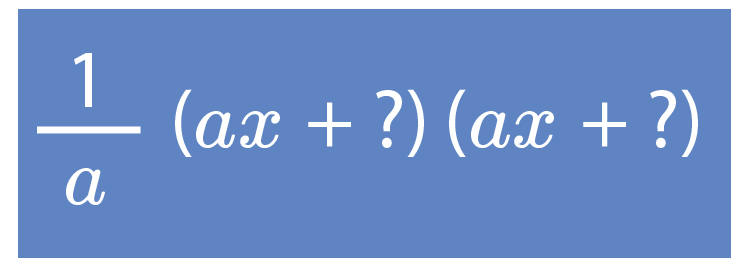We must ask

What two factors of
axxc

add to give b?

Example 1

Factorise the following quadratic 4x^2-5x-6=0

Find

What two factors of atimesc  add up to b

and in this case because atimesc=4times-6=-24

We must ask ourselves what factors of -24  add to give 5 :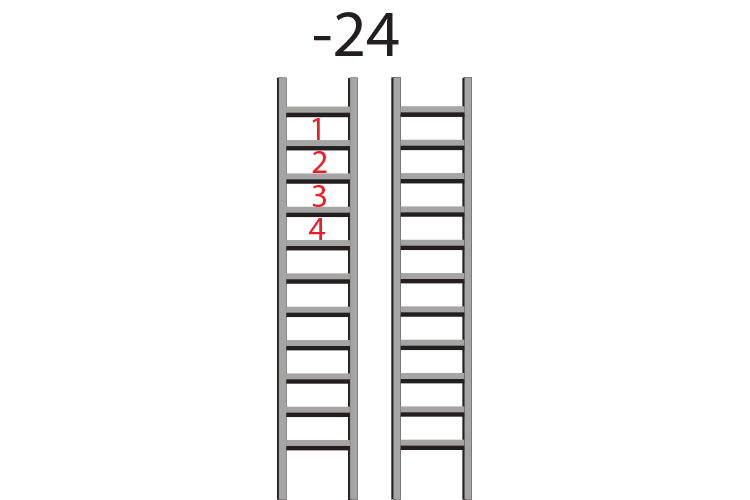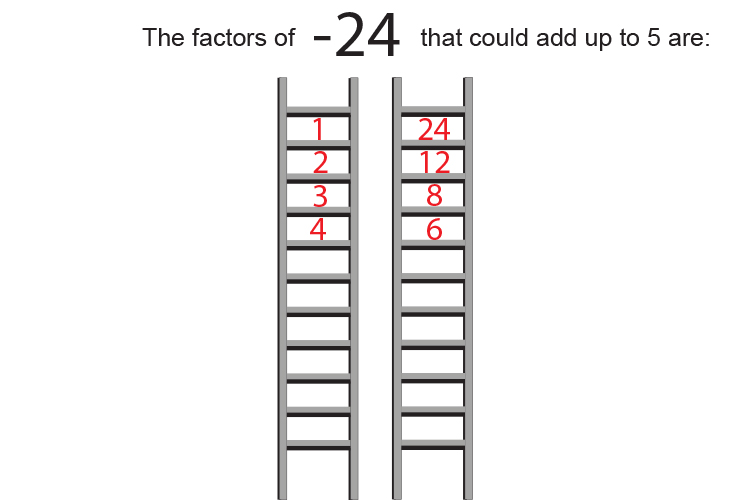The factors of -24  are:

 1 times -24 or -1 times 24 2 times -12 or -2 times 12 3 times -8 or -3 times 8 4 times -6 or -4 times 6

But the only two factors that add to give -5  are -8  and 3

Put this in the form

1/a(ax+?)(ax+?)

1/4(4x-8)(4x+3)

Pull out any multiples of 4 from the 1st set of brackets

1/4times4(1x-2)(4x+3)

1/(cancel4)timescancel4(1x-2)(4x+3)

(1x-2)(4x+3)

This can now be solved as

1x-2=0

and      4x+3=0

Which is the same as

x=2   or  x=(-3)/4

## Now check

If x=2                     4times2^2-5times2-6=0

16-10-6=0   Which is correct

If x=(-3)/4               4times((-3)/4)^2-5times(-3)/4-6=0

4times((-3)/4times(-3)/4)+15/4-6=0

4times9/16+15/4-6=0

36/16+15/4-6=0

2\4/16+3\3/4-6=0   Which is correct

Answer:

The roots of 4x^2-5x-6=0  are x=2   and  x=(-3)/4

Example 2

Factorise the following quadratic 6x^2+7x-3=0

Find

What two factors of atimesc  add up to give b

and in this case because atimesc=6times-3=-18

We must ask ourselves what factors of -18  add to give 7 :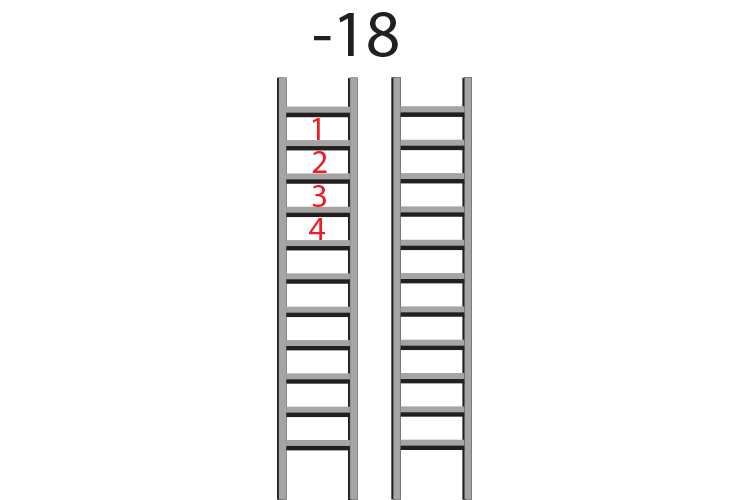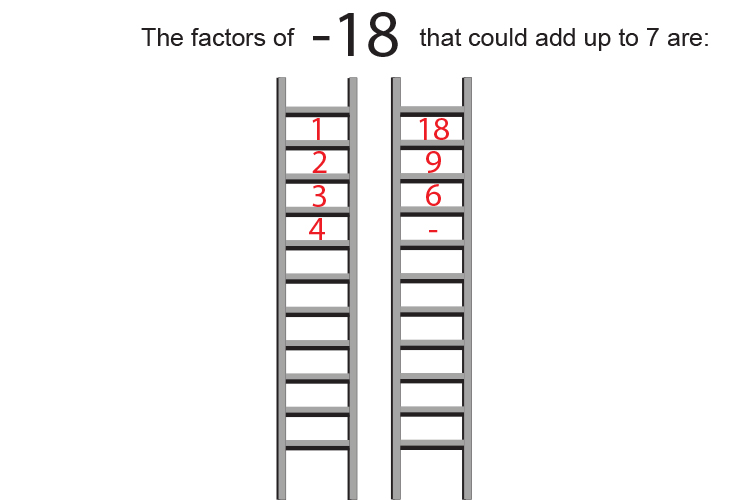The factors of -18  are:

 1 times -18 or -1 times 18 2 times -9 or -2 times 9 3 times -6 or -3 times 6

But the only two factors that add to give 7  are 9  and -2

Put this in the form

1/a(ax+?)(ax+?)

1/6(6x+9)(6x-2)

Pull out any multiples of 6 from the 1st set of brackets

1/6times6(1x+1.5)(6x-2)

1/cancel6timescancel6(1x+1.5)(6x-2)

(1x+3/2)(6x-2)

This can now be solved as

1x+3/2=0

and   6x-2=0

Which is

x=-3/2

and   x=2/6  or 1/3

## Now check

If x=-3/2             6times ((-3)/2)^2 +7times(-3)/2-3=0

6times((-3)/2times(-3)/2)-21/2-3=0

6times(9/4)-21/2-3=0

(6times9)/4-21/2-3=0

(3times9)/2-21/2-3=0

27/2-21/2-3=0

13\1/2-10\1/2-3=0   Which is correct

If x=1/3                   6times (1/3)^2 +7times1/3-3=0

6times(1/3times1/3)+7/3-3=0

6/9+7/3-3=0

2/3+2\1/3-3=0   Which is correct

Answer:

The roots of 6x^2+7x-3=0  are x=-3/2  and x=1/3

Example 3

Factorise the following quadratic 3x^2+14x-5=0

Find

What two factors of atimesc add up to b

and in this case because atimesc=3times-5=-15

We must ask ourselves what factors of -15  add to give 14 :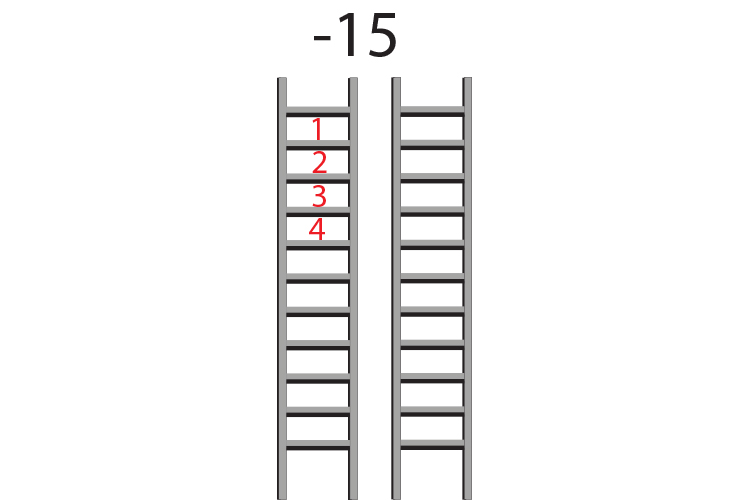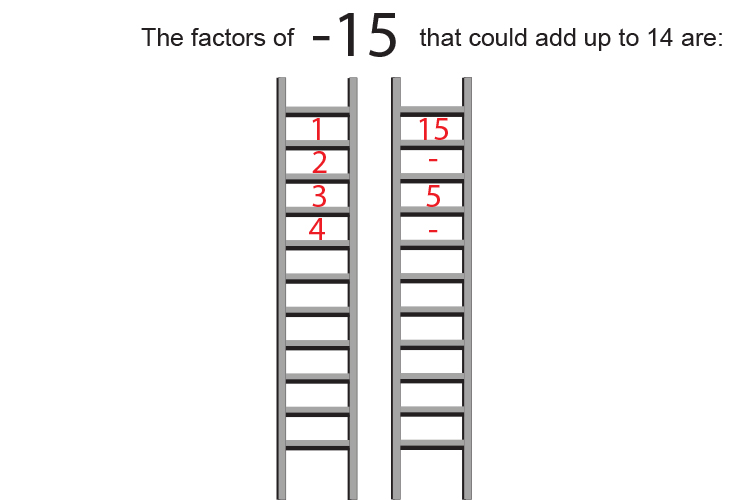The factors of -15  are:

 1 times -15 or -1 times 15 3 times -5 or -3 times 5

But the only two factors that add to give 14  are 15  and -1

Put this in the formula:

1/a(ax+?)(ax+?)

1/3(3x+15)(3x-1)

Pull out any multiples of 3 from the 1st set of brackets

1/3times3(1x+5)(3x-1)

1/cancel3timescancel3(1x+5)(3x-1)

This can now be solved as

1x+5=0

and    3x-1=0

Which is

x=-5

and    x=1/3

## Now check

If x=-5              3times (-5)^2 +14times(-5)-5=0

75-70-5=0   Which is correct

And if x=1/3           3times (1/3)^2 +14times(1/3)-5=0

3times (1/9) +14/3-5=0

3/9+14/3-5=0

1/3+4\2/3-5=0   Which is correct

Answer:

The roots of 3x^2+14x-5=0  are x=-5   and  x=1/3